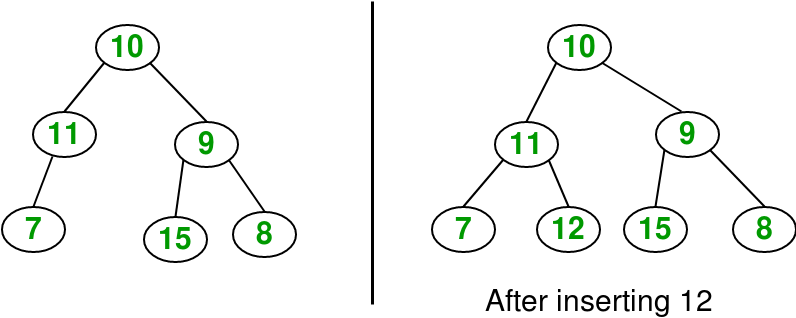Related Articles

# Insertion in a Binary Tree in level order

• Difficulty Level : Easy
• Last Updated : 31 Aug, 2021

Given a binary tree and a key, insert the key into the binary tree at the first position available in level order.The idea is to do iterative level order traversal of the given tree using queue. If we find a node whose left child is empty, we make new key as left child of the node. Else if we find a node whose right child is empty, we make the new key as right child. We keep traversing the tree until we find a node whose either left or right child is empty.

## C++

 `// C++ program to insert element in Binary Tree``#include ``#include ``using` `namespace` `std;` `/* A binary tree node has data, pointer to left child``and a pointer to right child */` `struct` `Node {``    ``int` `data;``    ``Node* left;``    ``Node* right;``};` `/* Function to create a new node */` `Node* CreateNode(``int` `data)``{``    ``Node* newNode = ``new` `Node();``    ``if` `(!newNode) {``        ``cout << ``"Memory error\n"``;``        ``return` `NULL;``    ``}``    ``newNode->data = data;``    ``newNode->left = newNode->right = NULL;``    ``return` `newNode;``}` `/* Function to insert element in binary tree */` `Node* InsertNode(Node* root, ``int` `data)``{``    ``// If the tree is empty, assign new node address to root``    ``if` `(root == NULL) {``        ``root = CreateNode(data);``        ``return` `root;``    ``}` `    ``// Else, do level order traversal until we find an empty``    ``// place, i.e. either left child or right child of some``    ``// node is pointing to NULL.``    ``queue q;``    ``q.push(root);` `    ``while` `(!q.empty()) {``        ``Node* temp = q.front();``        ``q.pop();` `        ``if` `(temp->left != NULL)``            ``q.push(temp->left);``        ``else` `{``            ``temp->left = CreateNode(data);``            ``return` `root;``        ``}` `        ``if` `(temp->right != NULL)``            ``q.push(temp->right);``        ``else` `{``            ``temp->right = CreateNode(data);``            ``return` `root;``        ``}``    ``}``}` `/* Inorder traversal of a binary tree */` `void` `inorder(Node* temp)``{``    ``if` `(temp == NULL)``        ``return``;` `    ``inorder(temp->left);``    ``cout << temp->data << ``' '``;``    ``inorder(temp->right);``}` `// Driver code``int` `main()``{``    ``Node* root = CreateNode(10);``    ``root->left = CreateNode(11);``    ``root->left->left = CreateNode(7);``    ``root->right = CreateNode(9);``    ``root->right->left = CreateNode(15);``    ``root->right->right = CreateNode(8);` `    ``cout << ``"Inorder traversal before insertion: "``;``    ``inorder(root);``    ``cout << endl;` `    ``int` `key = 12;``    ``root = InsertNode(root, key);` `    ``cout << ``"Inorder traversal after insertion: "``;``    ``inorder(root);``    ``cout << endl;` `    ``return` `0;``}`

## Java

 `// Java program to insert element in binary tree``import` `java.util.LinkedList;``import` `java.util.Queue;``public` `class` `GFG {` `    ``/* A binary tree node has key, pointer to``    ``left child and a pointer to right child */``    ``static` `class` `Node {``        ``int` `key;``        ``Node left, right;` `        ``// constructor``        ``Node(``int` `key)``        ``{``            ``this``.key = key;``            ``left = ``null``;``            ``right = ``null``;``        ``}``    ``}``    ``static` `Node root;``    ``static` `Node temp = root;` `    ``/* Inorder traversal of a binary tree*/``    ``static` `void` `inorder(Node temp)``    ``{``        ``if` `(temp == ``null``)``            ``return``;` `        ``inorder(temp.left);``        ``System.out.print(temp.key + ``" "``);``        ``inorder(temp.right);``    ``}` `    ``/*function to insert element in binary tree */``    ``static` `void` `insert(Node temp, ``int` `key)``    ``{` `        ``if` `(temp == ``null``) {``            ``root = ``new` `Node(key);``            ``return``;``        ``}``        ``Queue q = ``new` `LinkedList();``        ``q.add(temp);` `        ``// Do level order traversal until we find``        ``// an empty place.``        ``while` `(!q.isEmpty()) {``            ``temp = q.peek();``            ``q.remove();` `            ``if` `(temp.left == ``null``) {``                ``temp.left = ``new` `Node(key);``                ``break``;``            ``}``            ``else``                ``q.add(temp.left);` `            ``if` `(temp.right == ``null``) {``                ``temp.right = ``new` `Node(key);``                ``break``;``            ``}``            ``else``                ``q.add(temp.right);``        ``}``    ``}` `    ``// Driver code``    ``public` `static` `void` `main(String args[])``    ``{``        ``root = ``new` `Node(``10``);``        ``root.left = ``new` `Node(``11``);``        ``root.left.left = ``new` `Node(``7``);``        ``root.right = ``new` `Node(``9``);``        ``root.right.left = ``new` `Node(``15``);``        ``root.right.right = ``new` `Node(``8``);` `        ``System.out.print(``            ``"Inorder traversal before insertion:"``);``        ``inorder(root);` `        ``int` `key = ``12``;``        ``insert(root, key);` `        ``System.out.print(``            ``"\nInorder traversal after insertion:"``);``        ``inorder(root);``    ``}``}``// This code is contributed by Sumit Ghosh`

## Python3

 `# Python program to insert element in binary tree``class` `newNode():` `    ``def` `__init__(``self``, data):``        ``self``.key ``=` `data``        ``self``.left ``=` `None``        ``self``.right ``=` `None``        ` `""" Inorder traversal of a binary tree"""``def` `inorder(temp):` `    ``if` `(``not` `temp):``        ``return` `    ``inorder(temp.left)``    ``print``(temp.key,end ``=` `" "``)``    ``inorder(temp.right)`  `"""function to insert element in binary tree """``def` `insert(temp,key):` `    ``if` `not` `temp:``        ``root ``=` `newNode(key)``        ``return``    ``q ``=` `[]``    ``q.append(temp)` `    ``# Do level order traversal until we find``    ``# an empty place.``    ``while` `(``len``(q)):``        ``temp ``=` `q[``0``]``        ``q.pop(``0``)` `        ``if` `(``not` `temp.left):``            ``temp.left ``=` `newNode(key)``            ``break``        ``else``:``            ``q.append(temp.left)` `        ``if` `(``not` `temp.right):``            ``temp.right ``=` `newNode(key)``            ``break``        ``else``:``            ``q.append(temp.right)``    ` `# Driver code``if` `__name__ ``=``=` `'__main__'``:``    ``root ``=` `newNode(``10``)``    ``root.left ``=` `newNode(``11``)``    ``root.left.left ``=` `newNode(``7``)``    ``root.right ``=` `newNode(``9``)``    ``root.right.left ``=` `newNode(``15``)``    ``root.right.right ``=` `newNode(``8``)` `    ``print``(``"Inorder traversal before insertion:"``, end ``=` `" "``)``    ``inorder(root)` `    ``key ``=` `12``    ``insert(root, key)` `    ``print``()``    ``print``(``"Inorder traversal after insertion:"``, end ``=` `" "``)``    ``inorder(root)` `# This code is contributed by SHUBHAMSINGH10`

## C#

 `// C# program to insert element in binary tree``using` `System;``using` `System.Collections.Generic;` `class` `GFG {` `    ``/* A binary tree node has key, pointer to``    ``left child and a pointer to right child */``    ``public` `class` `Node {``        ``public` `int` `key;``        ``public` `Node left, right;` `        ``// constructor``        ``public` `Node(``int` `key)``        ``{``            ``this``.key = key;``            ``left = ``null``;``            ``right = ``null``;``        ``}``    ``}``    ``static` `Node root;` `    ``/* Inorder traversal of a binary tree*/``    ``static` `void` `inorder(Node temp)``    ``{``        ``if` `(temp == ``null``)``            ``return``;` `        ``inorder(temp.left);``        ``Console.Write(temp.key + ``" "``);``        ``inorder(temp.right);``    ``}` `    ``/*function to insert element in binary tree */``    ``static` `void` `insert(Node temp, ``int` `key)``    ``{``          ``if` `(temp == ``null``) {``            ``root = ``new` `Node(key);``            ``return``;``        ``}``        ``Queue q = ``new` `Queue();``        ``q.Enqueue(temp);` `        ``// Do level order traversal until we find``        ``// an empty place.``        ``while` `(q.Count != 0) {``            ``temp = q.Peek();``            ``q.Dequeue();` `            ``if` `(temp.left == ``null``) {``                ``temp.left = ``new` `Node(key);``                ``break``;``            ``}``            ``else``                ``q.Enqueue(temp.left);` `            ``if` `(temp.right == ``null``) {``                ``temp.right = ``new` `Node(key);``                ``break``;``            ``}``            ``else``                ``q.Enqueue(temp.right);``        ``}``    ``}` `    ``// Driver code``    ``public` `static` `void` `Main(String[] args)``    ``{``        ``root = ``new` `Node(10);``        ``root.left = ``new` `Node(11);``        ``root.left.left = ``new` `Node(7);``        ``root.right = ``new` `Node(9);``        ``root.right.left = ``new` `Node(15);``        ``root.right.right = ``new` `Node(8);` `        ``Console.Write(``            ``"Inorder traversal before insertion:"``);``        ``inorder(root);` `        ``int` `key = 12;``        ``insert(root, key);` `        ``Console.Write(``            ``"\nInorder traversal after insertion:"``);``        ``inorder(root);``    ``}``}` `// This code is contributed by Rajput-Ji`
Output

```Inorder traversal before insertion: 7 11 10 15 9 8
Inorder traversal after insertion: 7 11 12 10 15 9 8 ```

This article is contributed by Yash Singla. If you like GeeksforGeeks and would like to contribute, you can also write an article using write.geeksforgeeks.org or mail your article to review-team@geeksforgeeks.org. See your article appearing on the GeeksforGeeks main page and help other Geeks.